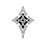# Interesting Trig sum

Prove/Disprove that

$\displaystyle \sum_{n=0}^{\infty} \left(\frac {\displaystyle\sum_{k=0}^{\left \lfloor \frac p2 \right\rfloor -1} \left(\cos \left(\frac {\pi}{p}(2k+1)(2n+1)\right)\right) }{(2n+1)^s}\right) =\left(\frac 12 -\frac {1}{2p^s }-\frac {\left\lfloor \frac p2\right\rfloor}{p^s}\right) (1-2^{-s})\zeta(s)$

Where $p$ is a prime number, $s\in R$ and $s\gt 1$

Also $\lfloor. \rfloor$ denotes the floor function while $\zeta(s)$ denotes the Riemann Zeta function.Note by Rohan Shinde
2 years, 4 months ago

This discussion board is a place to discuss our Daily Challenges and the math and science related to those challenges. Explanations are more than just a solution — they should explain the steps and thinking strategies that you used to obtain the solution. Comments should further the discussion of math and science.

When posting on Brilliant:

• Use the emojis to react to an explanation, whether you're congratulating a job well done , or just really confused .
• Ask specific questions about the challenge or the steps in somebody's explanation. Well-posed questions can add a lot to the discussion, but posting "I don't understand!" doesn't help anyone.
• Try to contribute something new to the discussion, whether it is an extension, generalization or other idea related to the challenge.

MarkdownAppears as
*italics* or _italics_ italics
**bold** or __bold__ bold
- bulleted- list
• bulleted
• list
1. numbered2. list
1. numbered
2. list
Note: you must add a full line of space before and after lists for them to show up correctly
paragraph 1paragraph 2

paragraph 1

paragraph 2

[example link](https://brilliant.org)example link
> This is a quote
This is a quote
    # I indented these lines
# 4 spaces, and now they show
# up as a code block.

print "hello world"
# I indented these lines
# 4 spaces, and now they show
# up as a code block.

print "hello world"
MathAppears as
Remember to wrap math in $$ ... $$ or $ ... $ to ensure proper formatting.
2 \times 3 $2 \times 3$
2^{34} $2^{34}$
a_{i-1} $a_{i-1}$
\frac{2}{3} $\frac{2}{3}$
\sqrt{2} $\sqrt{2}$
\sum_{i=1}^3 $\sum_{i=1}^3$
\sin \theta $\sin \theta$
\boxed{123} $\boxed{123}$

Sort by:

@Darkrai ~Rayquaza Any references???? I converted the sum into real parts of Polylogs but got stuck in finding the infinite summation.........

Basically, after a lot of simplifications, the problem breaks down to finding the real part of

$\displaystyle \left(\sum_{n=1}^{\infty}Li_s\left(a^{\left(2n-1\right)}\right)\right)-\frac{1}{2^s}\left(\sum_{n=1}^{\infty}Li_s\left(a^{\left(4n-2\right)}\right)\right)$

Here, $a$ is $\displaystyle e^{\frac{i\pi}{p}}$ and $\displaystyle Li_s\left(a\right)$ denotes the Polylogarithm of $a$ to the base $s$

- 2 years, 4 months ago